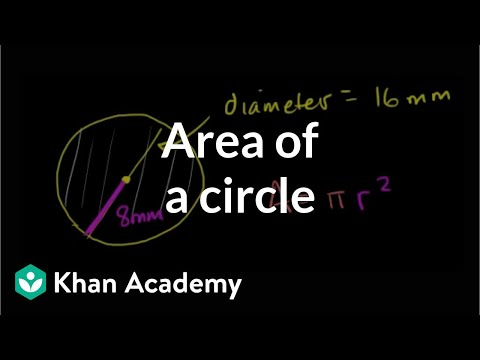Video

# Radius & diameter from circumference (Full video)

Description: Sal finds the radius and diameter of a circle given the circumference. Based on that, let's see if we can figure out what the radius of that same circle is going to be. We know that if its radius is of length r, that the circumference is going to be two pi times r. In fact, the number pi, the standard definition for it, is just the ratio between the circumference and the diameter of a circle. So, we could say 49 pi is going to be equal to two pi times the radius.

### Other videos you might be interested in### Area of a circle (Full video)